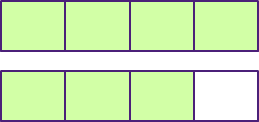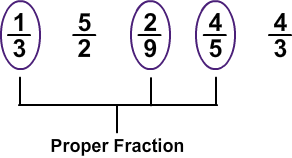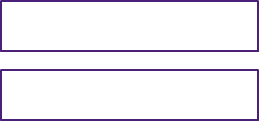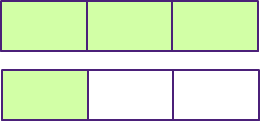# What is an Improper Fraction?

Print Rate 0 stars Common Core
Lesson size:
Message preview:
Someone you know has shared lesson with you:

To play this lesson, click on the link below:

https://www.turtlediary.com/lesson/improper-fractions.html

Hope you have a good experience with this site and recommend to your friends too.

Login to rate activities and track progress.
Login to rate activities and track progress.

## Proper and Improper Fractions

In a proper fraction, the numerator is smaller than the denominator.

Normal fractions are proper.

Example 1## Improper Fraction

In an improper fraction, the numerator is greater than the denominator.

They are called improper because if we illustrate the fraction on model, the number of shaded parts will be more than the total parts which is not practically possible. We can not distribute something in 13 parts if there are only 10 total parts.

### Example 2Let's take a closer look at the improper fraction
7
4
. If we try to draw a picture of it, we'll start by splitting a box into 4 pieces.But when we wish to color in 7 sections (because 7 is the numerator), we don't have enough sections to fill in. That means we actually need to draw a second box to fill in 7 sections.So,
7
4
is actually bigger than 1.That's true for all improper fractions.

### All improper fractions are larger than 1.

Let's illustrate through examples.

### Example 3

Which of these fractions are proper fractions?

1
3

5
2

2
9

4
5

4
3

### Solution

A proper fraction has a smaller numerator than denominator. Thus, the proper fractions are:### Example 4

Which of these fractions are improper fractions?

5
9

2
3

7
6

8
5

2
7

### Solution

An improper fraction has a larger numerator than denominator. Thus, the improper fractions are:### Example 5

Draw a picture of
4
3
.

### Solution

Since its an improper fraction, you'll need more than one box.Split both boxes into 3 pieces. Then color in 4 of the pieces.## What is an Improper Fraction?

• In a Proper Fraction, the numerator is smaller than the denominator.
• Normal fractions are proper fractions.
• In an Improper Fraction, the numerator is larger than the denominator.
• They are called improper because it is not the proper way to leave your answer.
• Improper fractions can be written as mixed numbers.

## Similar Lessons

Become premium member to get unlimited access.